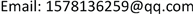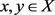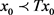﻿ 偏v-度量空间中有关Meir-Keeler-Rational型映射的不动点理论 Fixed Point Theorems for a Generalized Me-ir-Keeler-Rational Contraction in Partial v-Generalized Metric Spaces

Pure Mathematics
Vol. 09  No. 03 ( 2019 ), Article ID: 30358 , 10 pages
10.12677/PM.2019.93052

Fixed Point Theorems for a Generalized Meir-Keeler-Rational Contraction in Partial $\nu$ -Generalized Metric Spaces

Xiaohui Wang, Peisheng Ji, Fangyuan Dong

School of Mathematics and statistics, Qingdao University, Qingdao ShandongReceived: Apr. 26th, 2019; accepted: May 6th, 2019; published: May 22nd, 2019ABSTRACT

In this paper, we consider the ﬁxed points of generalized Meir-Keeler-Rational contractions in the context of partial $\nu$ -generalized metric spaces. Our results generalize several known ones in literature.

Keywords:Meir-Keeler-Rational Contraction, Partial $\nu$ -Generalized Metric Space, Fixed Points$\nu$ -度量空间中有关Meir-Keeler-Rational型 映射的不动点理论1. 引言

Banach  引入的Banach收缩原理是数学分析中最重要的结果之一，它是数学许多分支中应用最广泛的不动点理论，并在许多不同的方向上被进行了推广。其中一方面推广涉及度量空间的各种推广，且在新的框架中得到不动点的结果，例如，在b-度量空间、广义度量空间和偏序度量空间中发表了一些关于这个主题的论文。2014年，Shukla  推广了b-度量空间和偏序度量空间，定义了偏b-度量空间的概念。Mitrovic和Radenovic  推广了 $\nu$ -度量空间  ，引入了 ${b}_{\nu }\left(s\right)$ -度量空间的概念。Abdullahi和Kumam  推广了偏序度量空间和 ${b}_{\nu }\left(s\right)$ -度量空间，定义了偏 ${b}_{\nu }\left(s\right)$ -度量空间，且在其中建立了不动点理论。他们在论文中提出了一些开放型问题：是否可以类似的在推广的 $\nu$ -度量空间， ${b}_{\nu }\left(s\right)$ -度量空间，偏 ${b}_{\nu }\left(s\right)$ -度量空间中证明Chatterjee型压缩、Hardy-Roger型压缩、Ciric型压缩和Suzuki型压缩的不动点问题。

$\epsilon \le d\left(x,y\right)<\epsilon +\delta ⇒d\left(Ax,Ay\right)<\epsilon$

1) 对给定的 $\epsilon >0$，存在 ，对于任意的 $x,y\in X$，有

$\epsilon

$m\left(x,y\right)=\mathrm{max}\left\{d\left(x,y\right),d\left(x,Ax\right),d\left(y,Ay\right),\left[d\left(x,Ay\right)+d\left(y,Ax\right)\right]/2\right\}$ ;

2) 对任意的 $x,y\in X$，且 $m\left(x,y\right)>0$

$d\left(Ax,Ay\right)

2. 预备知识

1) $x=y$ 当且仅当 $d\left(x,y\right)=d\left(x,x\right)=d\left(y,y\right)$

2) $d\left(x,x\right)\le d\left(x,y\right)$

3) $d\left(x,y\right)=d\left(y,x\right)$

4) 存在 $s\in R$$s\ge 1$，有 $d\left(x,y\right)\le s\left[d\left(x,{u}_{1}\right)+d\left({u}_{1},{u}_{2}\right)+\cdots +d\left({u}_{\nu },y\right)\right]-{\sum }_{k=1}^{\nu }d\left({u}_{k},{u}_{k}\right)$ (偏 ${b}_{\nu }\left(s\right)$ -不等式)。

1) 偏 ${b}_{1}\left(1\right)$ -度量空间是偏度量空间  ；

2) 偏 ${b}_{1}\left(s\right)$ -度量空间是偏b-度量空间，系数 $s\ge 1$  ；

3) 偏 ${b}_{2}\left(1\right)$ -度量空间是偏矩形度量空间  ；

4) 偏 ${b}_{2}\left(s\right)$ -度量空间是偏矩形b-度量空间，系数 $s\ge 1$

5) 偏 ${b}_{\nu }\left(1\right)$ -度量空间是偏 $\nu$ -度量空间。

1) $x=y$ 当且仅当 $d\left(x,y\right)=d\left(x,x\right)=d\left(y,y\right)$

2) $d\left(x,x\right)\le d\left(x,y\right)$

3) $d\left(x,y\right)=d\left(y,x\right)$

4) $d\left(x,y\right)\le d\left(x,{u}_{1}\right)+d\left({u}_{1},{u}_{2}\right)+\cdots +d\left({u}_{\nu },y\right)-{\sum }_{k=1}^{\nu }d\left({u}_{k},{u}_{k}\right)$ (偏 $\nu$ -不等式)。

1) 若 $\underset{n\to \infty }{\mathrm{lim}}d\left({x}_{n},x\right)=d\left(x,x\right)$，则数列 $\left\{{x}_{n}\right\}$ 称为X中的收敛列，且收敛于x。x称为数列 $\left\{{x}_{n}\right\}$ 的极限，记为 $\underset{n\to \infty }{\mathrm{lim}}{x}_{n}=x$，或 ${x}_{n}\to x$$n\to \infty$

2) 若 $\underset{n,m\to \infty }{\mathrm{lim}}d\left({x}_{n},{x}_{m}\right)$ 存在，则数列 $\left\{{x}_{n}\right\}$ 称为X中的柯西列。

3) 若对于X中的每个柯西列 $\left\{{x}_{n}\right\}$，存在 $x\in X$，且 $\underset{n,m\to \infty }{\mathrm{lim}}d\left({x}_{n},{x}_{m}\right)=\underset{n,m\to \infty }{\mathrm{lim}}d\left({x}_{n},x\right)=d\left(x,x\right)$，则 $\left(X,d\right)$ 称为完备的偏 ${b}_{\nu }\left(s\right)$ -度量空间。

Samet等人  通过定义 $\alpha -\psi$ -收缩映射和 $\alpha$ -容许映射给出了一个有趣的结果，同时也推广了Banach收缩原理。

$\alpha \left(x,y\right)\ge 1⇒\alpha \left(Tx,Ty\right)\ge 1$

1) 对任意 $x,y\in X$，且 $\alpha \left(x,y\right)\ge 1$，有 $\alpha \left(Tx,Ty\right)\ge 1$ 成立。

2) 对任意的 $x,y,z\in X$，且 $\alpha \left(x,z\right)\ge 1$$\alpha \left(z,y\right)\ge 1$，有 $\alpha \left(x,y\right)\ge 1$ 成立。

$\underset{n,m\to \infty }{\mathrm{lim}}d\left({x}_{n},{x}_{m}\right)=\underset{n,m\to \infty }{\mathrm{lim}}d\left({x}_{n},x\right)=d\left(x,x\right)=\underset{n\to \infty }{\mathrm{lim}}d\left({x}_{n},y\right)=d\left(y,y\right)$

$\begin{array}{c}d\left(x,y\right)\le d\left(x,{x}_{n+1}\right)+d\left({x}_{n+1},{x}_{n+2}\right)+\cdots +d\left({x}_{n+\nu -1},{x}_{n+\nu }\right)+d\left({x}_{n+\nu },y\right)-\underset{k=1}{\overset{\nu }{\sum }}d\left({x}_{n+k},{x}_{n+k}\right)\\ \le d\left(x,{x}_{n+1}\right)+d\left({x}_{n+1},{x}_{n+2}\right)+\cdots +d\left({x}_{n+\nu -1},{x}_{n+\nu }\right)+d\left({x}_{n+\nu },y\right)\end{array}$

3. 主要结论

1) 对所有的，且 $\alpha \left(x,y\right)\ge 1,M\left(x,y\right)>0$，有

$d\left(Tx,Ty\right) (3.1)

$M\left(x,y\right)=\mathrm{max}\left\{d\left(x,y\right),d\left(x,Tx\right),d\left(y,Ty\right),\frac{d\left(x,Tx\right)d\left(y,Ty\right)}{1+d\left(x,y\right)+d\left(x,Ty\right)+d\left(y,Tx\right)},\frac{d\left(x,Tx\right)d\left(y,Ty\right)}{1+d\left(Tx,Ty\right)}\right\}$ ;

2) 对任意的 $\epsilon >0$，存在 $\delta >0$，对所有的 $x,y\in X$，有

$\alpha \left(x,y\right)\ge 1$ , $M\left(x,y\right)<\epsilon +\delta ⇒d\left(Tx,Ty\right)\le \epsilon$ ; (3.2)

3) T是三角 $\alpha$ -容许映射，且 $\alpha$ 具有反身性；

4) 存在 ${x}_{0}\in X$，有 $\alpha \left({x}_{0},T{x}_{0}\right)\ge 1$ 成立；

5) T是连续的。

$\alpha \left({x}_{n},{x}_{m}\right)\ge 1$ (3.3)

1) 我们首先证明 $\underset{n\to \infty }{\mathrm{lim}}d\left({x}_{n-1},{x}_{n}\right)=0$

$d\left({x}_{n},{x}_{n+1}\right)=d\left(T{x}_{n-1},T{x}_{n}\right) (3.4)

$\begin{array}{l}M\left({x}_{n-1},{x}_{n}\right)\\ =\mathrm{max}\left\{d\left({x}_{n-1},{x}_{n}\right),d\left({x}_{n-1},T{x}_{n-1}\right),d\left({x}_{n},T{x}_{n}\right),\frac{d\left({x}_{n-1},T{x}_{n-1}\right)d\left({x}_{n},T{x}_{n}\right)}{1+d\left({x}_{n-1},{x}_{n}\right)+d\left({x}_{n-1},T{x}_{n}\right)+d\left({x}_{n},T{x}_{n-1}\right)},\\ \text{\hspace{0.17em}}\text{\hspace{0.17em}}\text{\hspace{0.17em}}\frac{d\left({x}_{n-1},T{x}_{n-1}\right)d\left({x}_{n},T{x}_{n}\right)}{1+d\left(T{x}_{n-1},T{x}_{n}\right)}\right\}\\ =\mathrm{max}\left\{d\left({x}_{n-1},{x}_{n}\right),d\left({x}_{n-1},{x}_{n}\right),d\left({x}_{n},{x}_{n+1}\right),\frac{d\left({x}_{n-1},{x}_{n}\right)d\left({x}_{n},{x}_{n+1}\right)}{1+d\left({x}_{n-1},{x}_{n}\right)+d\left({x}_{n-1},{x}_{n+1}\right)},\frac{d\left({x}_{n-1},{x}_{n}\right)d\left({x}_{n},{x}_{n+1}\right)}{1+d\left({x}_{n},{x}_{n+1}\right)}\right\}\\ =\mathrm{max}\left\{d\left({x}_{n-1},{x}_{n}\right),d\left({x}_{n},{x}_{n+1}\right)\right\}\end{array}$ (3.5)

$d\left({x}_{n},{x}_{n+1}\right) (3.6)

$\alpha \left(x,y\right)\ge 1$ , $M\left(x,y\right)<\epsilon +\delta ⇒d\left(Tx,Ty\right)\le \epsilon$ (3.7)

$M\left({x}_{n},{x}_{n+1}\right)=d\left({x}_{n},{x}_{n+1}\right)

$\underset{n\to \infty }{\mathrm{lim}}d\left({x}_{n},{x}_{n+1}\right)=0$ (3.8)

2) 下面我们证明对所有的 $n\ne m$，有 ${x}_{n}\ne {x}_{m}$。假设若对某一 $n>m$，有 ${x}_{n}={x}_{m}$ 成立，因此我们得到

${x}_{n+1}=T{x}_{n}=T{x}_{m}={x}_{m+1}$。由(3.6)，得到 $d\left({x}_{n},{x}_{n+1}\right)，矛盾。因此，对任意 $n\ne m$${x}_{n}\ne {x}_{m}$

3) 接下来证明，对任意的 $p\in N$，且 $p\ge 2$，有 $\underset{n\to \infty }{\mathrm{lim}}d\left({x}_{n},{x}_{n+p}\right)=0$ 成立。

$d\left({x}_{n},{x}_{n+p}\right) (3.9)

$\begin{array}{l}M\left({x}_{n-1},{x}_{n+p-1}\right)\\ =\mathrm{max}\left\{d\left({x}_{n-1},{x}_{n+p-1}\right),d\left({x}_{n-1},T{x}_{n-1}\right),d\left({x}_{n+p-1},T{x}_{n+p-1}\right),\begin{array}{c}\text{ }\\ \text{ }\end{array}\\ \text{\hspace{0.17em}}\text{\hspace{0.17em}}\frac{d\left({x}_{n-1},T{x}_{n-1}\right)d\left({x}_{n+p-1},T{x}_{n+p-1}\right)}{1+d\left({x}_{n-1},{x}_{n+p-1}\right)+d\left({x}_{n-1},T{x}_{n+p-1}\right)+d\left(T{x}_{n-1},{x}_{n+p-1}\right)},\frac{d\left({x}_{n-1},T{x}_{n-1}\right)d\left({x}_{n+p-1},T{x}_{n+p-1}\right)}{1+d\left(T{x}_{n-1},T{x}_{n+p-1}\right)}\right\}\\ =\mathrm{max}\left\{d\left({x}_{n-1},{x}_{n+p-1}\right),d\left({x}_{n-1},{x}_{n}\right),d\left({x}_{n+p-1},{x}_{n+p}\right),\begin{array}{c}\text{ }\\ \text{ }\end{array}\\ \text{\hspace{0.17em}}\text{\hspace{0.17em}}\frac{d\left({x}_{n-1},{x}_{n}\right)d\left({x}_{n+p-1},{x}_{n+p}\right)}{1+d\left({x}_{n-1},{x}_{n+p-1}\right)+d\left({x}_{n-1},{x}_{n+p}\right)+d\left({x}_{n},{x}_{n+p-1}\right)},\frac{d\left({x}_{n-1},{x}_{n}\right)d\left({x}_{n+p-1},{x}_{n+p}\right)}{1+d\left({x}_{n},{x}_{n+p}\right)}\right\}\end{array}$

$M\left({x}_{n-1},{x}_{n+p-1}\right)=\mathrm{max}\left\{d\left({x}_{n-1},{x}_{n+p-1}\right),d\left({x}_{n-1},{x}_{n}\right)\right\}$ (3.10)

$d\left({x}_{n},{x}_{n+p}\right)<\mathrm{max}\left\{d\left({x}_{n-1},{x}_{n+p-1}\right),d\left({x}_{n-1},{x}_{n}\right)\right\}$ (3.11)

${a}_{n}=d\left({x}_{n},{x}_{n+p}\right)$${b}_{n}=d\left({x}_{n},{x}_{n+1}\right)$，故

${a}_{n}<\mathrm{max}\left\{{a}_{n-1},{b}_{n-1}\right\}$

$\underset{n\to \infty }{\mathrm{lim}}{a}_{n}=\underset{n\to \infty }{\mathrm{lim}}\mathrm{max}\left\{{a}_{n},{b}_{n}\right\}=t$

$t>0$，因为 $\underset{n\to \infty }{\mathrm{lim}}{b}_{n}=0$$\underset{n\to \infty }{\mathrm{lim}}{a}_{n}=\underset{n\to \infty }{\mathrm{lim}}\mathrm{max}\left\{{a}_{n},{b}_{n}\right\}=t>0$，接下来，我们假设 ${a}_{n}>{b}_{n}$$n\in N$，即 ${a}_{n}=\mathrm{max}\left\{{a}_{n},{b}_{n}\right\}>t$$n\in N$

$\epsilon =t$，存在 $\delta >0$，对任意的 $x,y\in X$，有

$\alpha \left(x,y\right)\ge 1$ , $M\left(x,y\right)<\epsilon +\delta ⇒d\left(Tx,Ty\right)\le \epsilon$ (3.12)

$M\left({x}_{n},{x}_{n+p}\right)=d\left({x}_{n},{x}_{n+p}\right)

$\underset{n\to \infty }{\mathrm{lim}}d\left({x}_{n},{x}_{n+p}\right)=0$ (3.13)

4) 下面我们证明 $\underset{n,m\to \infty }{\mathrm{lim}}d\left({x}_{n},{x}_{m}\right)=0$。相反地，我们假设 $\left\{d\left({x}_{n},{x}_{m}\right)\right\}$ 不收敛于0，选取子列 $\left\{{x}_{{m}_{i}}\right\},\left\{{x}_{{n}_{i}}\right\}\subset \left\{{x}_{n}\right\}$，存在 ${\epsilon }_{0}>0$${n}_{i}$ 是使下列式子成立的最小指标

 , $d\left({x}_{{m}_{i}},{x}_{{n}_{i}}\right)>2{\epsilon }_{0}$ (3.14)

$\alpha \left(x,y\right)\ge 1$ , $M\left(x,y\right)<{\epsilon }_{0}+\delta ⇒d\left(Tx,Ty\right)\le {\epsilon }_{0}$ (3.15)

$d\left({x}_{n},{x}_{n+p}\right)<\frac{{\delta }^{\prime }}{3\nu }$ (3.16)

$j\in \left[{m}_{{i}_{0}},{n}_{{i}_{0}}\right]$，由偏 $\nu$ -不等式，有

$\begin{array}{c}d\left({x}_{{m}_{{i}_{0}}},{x}_{j}\right)\le d\left({x}_{{m}_{{i}_{0}}},{x}_{j+1}\right)+d\left({x}_{j+1},{x}_{j+2}\right)+\cdots +d\left({x}_{j+\nu -1},{x}_{j+\nu }\right)+d\left({x}_{j+\nu },{x}_{j}\right)-\underset{k=1}{\overset{\nu }{\sum }}d\left({x}_{j+k},{x}_{j+k}\right)\\ \le d\left({x}_{{m}_{{i}_{0}}},{x}_{j+1}\right)+\nu ×\frac{{\delta }^{\prime }}{3\nu }=d\left({x}_{{m}_{{i}_{0}}},{x}_{j+1}\right)+\frac{{\delta }^{\prime }}{3}\end{array}$

$\begin{array}{c}d\left({x}_{{m}_{{i}_{0}}},{x}_{j+1}\right)\le d\left({x}_{{m}_{{i}_{0}}},{x}_{j}\right)+d\left({x}_{j},{x}_{j+2}\right)+\cdots +d\left({x}_{j+\nu -1},{x}_{j+\nu }\right)\\ +d\left({x}_{j+\nu },{x}_{j+1}\right)-d\left({x}_{j},{x}_{j}\right)-\underset{k=2}{\overset{\nu }{\sum }}d\left({x}_{j+k},{x}_{j+k}\right)\\ \le d\left({x}_{{m}_{{i}_{0}}},{x}_{j}\right)+\nu ×\frac{{\delta }^{\prime }}{3\nu }=d\left({x}_{{m}_{{i}_{0}}},{x}_{j}\right)+\frac{{\delta }^{\prime }}{3}\end{array}$

$|d\left({x}_{{m}_{{i}_{0}}},{x}_{j}\right)-d\left({x}_{{m}_{{i}_{0}}},{x}_{j+1}\right)|\le \frac{{\delta }^{\prime }}{3}$

${\epsilon }_{0}+\frac{2{\delta }^{\prime }}{3} (3.17)

$\begin{array}{l}M\left({x}_{{m}_{{i}_{0}}},{x}_{{j}_{0}}\right)\\ =\mathrm{max}\left\{d\left({x}_{{m}_{{i}_{0}}},{x}_{{j}_{0}}\right),d\left({x}_{{m}_{{i}_{0}}},T{x}_{{m}_{{i}_{0}}}\right),d\left({x}_{{j}_{0}},T{x}_{{j}_{0}}\right),\begin{array}{c}\text{ }\\ \text{ }\\ \text{ }\end{array}\\ \text{\hspace{0.17em}}\text{\hspace{0.17em}}\text{ }\frac{d\left({x}_{{m}_{{i}_{0}}},T{x}_{{m}_{{i}_{0}}}\right)d\left({x}_{{j}_{0}},T{x}_{{j}_{0}}\right)}{1+d\left({x}_{{m}_{{i}_{0}}},{x}_{{j}_{0}}\right)+d\left({x}_{{m}_{{i}_{0}}},T{x}_{{j}_{0}}\right)+d\left(T{x}_{{m}_{{i}_{0}}},{x}_{{j}_{0}}\right)},\frac{d\left({x}_{{m}_{{i}_{0}}},T{x}_{{m}_{{i}_{0}}}\right)d\left({x}_{{j}_{0}},T{x}_{{j}_{0}}\right)}{1+d\left(T{x}_{{m}_{{i}_{0}}},T{x}_{{j}_{0}}\right)}\right\}\\ =\mathrm{max}\left\{d\left({x}_{{m}_{{i}_{0}}},{x}_{{j}_{0}}\right),d\left({x}_{{m}_{{i}_{0}}},{x}_{{m}_{{i}_{0}}+1}\right),d\left({x}_{{j}_{0}},{x}_{{j}_{0}+1}\right),\begin{array}{c}\text{ }\\ \text{ }\\ \text{ }\end{array}\\ \text{\hspace{0.17em}}\text{\hspace{0.17em}}\text{ }\frac{d\left({x}_{{m}_{{i}_{0}}},{x}_{{m}_{{i}_{0}}+1}\right)d\left({x}_{{j}_{0}},{x}_{{j}_{0}+1}\right)}{1+d\left({x}_{{m}_{{i}_{0}}},{x}_{{j}_{0}}\right)+d\left({x}_{{m}_{{i}_{0}}},{x}_{{j}_{0}+1}\right)+d\left({x}_{{m}_{{i}_{0}}+1},{x}_{{j}_{0}}\right)},\frac{d\left({x}_{{m}_{{i}_{0}}},{x}_{{m}_{{i}_{0}}+1}\right)d\left({x}_{{j}_{0}},{x}_{{j}_{0}+1}\right)}{1+d\left({x}_{{m}_{{i}_{0}}+1},T{x}_{{j}_{0}+1}\right)}\right\}\\ =d\left({x}_{{m}_{{i}_{0}}},{x}_{{j}_{0}}\right)\end{array}$ (3.18)

$\begin{array}{c}{\epsilon }_{0}+\frac{2{\delta }^{\prime }}{3}

$\underset{n,m\to \infty }{\mathrm{lim}}d\left({x}_{n},{x}_{m}\right)=\underset{n\to \infty }{\mathrm{lim}}d\left({x}_{n},u\right)=d\left(u,u\right)=0$

5) 假设T是连续的，下面我们证明u是T的不动点。因为T是连续的，则

$\underset{n\to \infty }{\mathrm{lim}}d\left(T{x}_{n},Tu\right)=\underset{n\to \infty }{\mathrm{lim}}d\left({x}_{n+1},Tu\right)=d\left(Tu,Tu\right)$

$\begin{array}{c}d\left(u,Tu\right)\le d\left(u,{x}_{n+\nu }\right)+d\left({x}_{n+\nu },{x}_{n+\nu -1}\right)+\cdots +d\left({x}_{n+2},{x}_{n+1}\right)+d\left({x}_{n+1},Tu\right)-\underset{k=1}{\overset{\nu }{\sum }}d\left({x}_{n+k},{x}_{n+k}\right)\\ \le d\left(u,{x}_{n+\nu }\right)+d\left({x}_{n+\nu },{x}_{n+\nu -1}\right)+\cdots +d\left({x}_{n+2},{x}_{n+1}\right)+d\left({x}_{n+1},Tu\right)\end{array}$

$\underset{n,m\to \infty }{\mathrm{lim}}d\left({x}_{n},{x}_{m}\right)=0$，得到 $d\left(u,Tu\right)\le d\left(Tu,Tu\right)$。因此

$d\left(u,Tu\right)=d\left(Tu,Tu\right)$ (3.19)

$\begin{array}{c}M\left(u,u\right)=\mathrm{max}\left\{d\left(u,u\right),d\left(u,Tu\right),d\left(u,Tu\right),\frac{d\left(u,Tu\right)d\left(u,Tu\right)}{1+d\left(u,u\right)+d\left(u,Tu\right)+d\left(u,Tu\right)},\frac{d\left(u,Tu\right)d\left(u,Tu\right)}{1+d\left(Tu,Tu\right)}\right\}\\ =d\left(u,Tu\right)>0\end{array}$

$d\left(Tu,Tu\right)

6) 最后，我们证明T的不动点是唯一的。假设 $u,v$ 是T的两个不动点，且 $u\ne v$。则由假设 $\alpha \left(u,v\right)\ge 1$

$d\left(u,v\right)=d\left(Tu,Tv\right)

$M\left(u,v\right)=\mathrm{max}\left\{d\left(u,v\right),d\left(u,Tu\right),d\left(v,Tv\right),\frac{d\left(u,Tu\right)d\left(v,Tv\right)}{1+d\left(u,v\right)+d\left(u,Tv\right)+d\left(v,Tu\right)},\frac{d\left(u,Tu\right)d\left(v,Tv\right)}{1+d\left(Tu,Tv\right)}\right\}=d\left(u,v\right)$

$d\left(u,v\right)

1) 对任意的 $x,y\in X$，且 $x\prec y$，若 $M\left(x,y\right)>0$，则有 $d\left(Tx,Ty\right)，其中

$M\left(x,y\right)=\mathrm{max}\left\{d\left(x,y\right),d\left(x,Tx\right),d\left(y,Ty\right),\frac{d\left(x,Tx\right)d\left(y,Ty\right)}{1+d\left(x,y\right)+d\left(x,Ty\right)+d\left(y,Tx\right)},\frac{d\left(x,Tx\right)d\left(y,Ty\right)}{1+d\left(Tx,Ty\right)}\right\}$ ;

2) 对任意的 $\epsilon >0$，存在 $\delta >0$，且对任意的 $x,y\in X$，有

$x\prec y$ , $M\left(x,y\right)<\epsilon +\delta ⇒d\left(Tx,Ty\right)\le \epsilon$ ;

3) 存在 ${x}_{0}\in X$，满足4) T是连续的。

$\alpha \left(x,y\right)=\left\{\begin{array}{l}1\text{\hspace{0.17em}}\text{\hspace{0.17em}}\text{\hspace{0.17em}}\text{\hspace{0.17em}}\text{\hspace{0.17em}}\text{\hspace{0.17em}}x\prec y\\ 0\text{\hspace{0.17em}}\text{\hspace{0.17em}}\text{\hspace{0.17em}}\text{\hspace{0.17em}}\text{\hspace{0.17em}}其他\end{array}$

Fixed Point Theorems for a Generalized Me-ir-Keeler-Rational Contraction in Partial v-Generalized Metric Spaces[J]. 理论数学, 2019, 09(03): 393-402. https://doi.org/10.12677/PM.2019.93052

1. 1. Banach, S. (1922) Sur les operations dans les ensembles abstraits et leur application aux equations integrals. Fundamenta Mathematicae, 20, 133-181.
https://doi.org/10.4064/fm-3-1-133-181

2. 2. Shukla, S. (2014) Partial b-Metric Spaces and Fixed Point Theorems. Mediterranean Journal of Mathematics, 11, 703-711.
https://doi.org/10.1007/s00009-013-0327-4

3. 3. Mitrovic, Z.D. and Radenovic, S. (2017) The Banach and Reich Contractions in -Metric Spaces. Fixed Point Theory and Applications, 19, 3087-3095.
https://doi.org/10.1007/s11784-017-0469-2

4. 4. Branciari, A. (2000) A Fixed Point Theorem of Banach-Caccioppoli Type on a Class of Generalized Metric Spaces. Publicationes Mathematicae, 57, 31-37.

5. 5. Abdullahi, M.S. and Kumam, P. (2018) Partial -Metric Spaces and Fixed Point Theorems. Fixed Point Theory and Applications, 20, 113.
https://doi.org/10.1007/s11784-018-0591-9

6. 6. Meir, A. and Keeler, E. (1969) A Theorem on Contraction Mappings. Journal of Mathematical Analysis & Applications, 28, 326-329.
https://doi.org/10.1016/0022-247x(69)90031-6

7. 7. Jachymski, J. (1995) Equivalent Conditions and the Meir-Keeler Type Theorems. Journal of Mathematical Analysis & Applications, 194, 293-303.
https://doi.org/10.1006/jmaa.1995.1299

8. 8. Mattehews, S.G. (1994) Partial Metric Topology. Annals of the New York Academy of Sciences, 728, 183-197.

9. 9. Shukla, S. (2014) Partial Rectangular Metric Spaces and Fixed Point Theorems. The Scientific World Journal, 2014, Article ID: 756298.
https://doi.org/10.1155/2014/756298

10. 10. Suzuki, T. (2014) Generalized Metric Spaces Do Not Have the Compatible Topology. Abstract and Applied Analysis, 2014, Article ID: 458098.
https://doi.org/10.1155/2014/458098

11. 11. Samet, B., Vetro, C. and Vetro, P. (2012) Fixed Point Theorems for -Contractive Type Mappings. Nonlinear Analysis, 75, 2154-2165.
https://doi.org/10.1016/j.na.2011.10.014

12. 12. Vahid, P., Nawab, H. and Zoran, K. (2016) Generalized Wardowski Type Fixed Point Theorems via -Admissable FG-Contractions in b-Metric Spaces. Acta Mathematica Scientia, 5, 1445-1456.
https://doi.org/10.1016/s0252-9602(16)30080-7